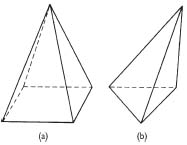Definition: pyramid from Philip's Encyclopedia

In geometry, solid figure having a polygon as one of its faces (the base), the other faces being triangles with a common vertex. Its volume is one third of the base area times the vertical height. Pyramids are described by the shape of their bases, such as a square pyramid or a triangular pyramid.

Summary Article: pyramid
from The Penguin Dictionary of Mathematics

A solid figure (a polyhedron) formed by a polygon (the base) and a number of triangles (lateral faces) with a common vertex that is not coplanar with the base. Line segments from the common vertex to the vertices of the base are lateral edges of the pyramid. Pyramids are named according to the base: a triangular pyramid (which is a tetrahedron), a square pyramid, a pentagonal pyramid, etc.

If the base has a centre, a line from the centre to the vertex is the axis of the pyramid. A pyramid that has its axis perpendicular to its base is a right pyramid; otherwise, it is an oblique pyramid. If the base is a regular polygon and the pyramid is a right pyramid, then it is also a regular pyramid.

The altitude (h) of a pyramid is the perpendicular distance from the base to the vertex. The volume of any pyramid is 1/3 Ah, where A is the area of the base. In a regular pyramid, all the lateral edges have the same length. The slant height (s) of the pyramid is the altitude of a face; the total surface area of the lateral faces is 1/2sp, where p is the perimeter of the base polygon.pyramid (a) Right square and (b) oblique triangular pyramids.Copyright © Penguin Books Ltd, 1989, 1998, 2003, 2008

Related Articles

Full text Article pyramid
Word Origins

[16 century] Egypt seems a likely ultimate source for pyramid , but its earliest known ancestor is Greek puramís , which passed into...

See more from Credo